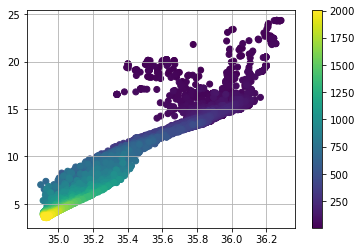# Numpy and Matplotlib¶

These are two of the most fundamental parts of the scientific python "ecosystem". Most everything else is built on top of them.

In :
import numpy as np


What did we just do? We imported a package. This brings new variables (mostly functions) into our interpreter. We access them as follows.

In :
# find out what is in our namespace
dir()

Out:
['In',
'Out',
'_',
'__',
'___',
'__builtin__',
'__builtins__',
'__doc__',
'__loader__',
'__name__',
'__package__',
'__spec__',
'_dh',
'_i',
'_i1',
'_i2',
'_ih',
'_ii',
'_iii',
'_oh',
'_sh',
'exit',
'get_ipython',
'np',
'quit']
In :
# find out what's in numpy
dir(np)

Out:
['ALLOW_THREADS',
'BUFSIZE',
'CLIP',
'ComplexWarning',
'DataSource',
'ERR_CALL',
'ERR_DEFAULT',
'ERR_IGNORE',
'ERR_LOG',
'ERR_PRINT',
'ERR_RAISE',
'ERR_WARN',
'FLOATING_POINT_SUPPORT',
'FPE_DIVIDEBYZERO',
'FPE_INVALID',
'FPE_OVERFLOW',
'FPE_UNDERFLOW',
'False_',
'Inf',
'Infinity',
'MAXDIMS',
'MAY_SHARE_BOUNDS',
'MAY_SHARE_EXACT',
'MachAr',
'ModuleDeprecationWarning',
'NAN',
'NINF',
'NZERO',
'NaN',
'PINF',
'PZERO',
'PackageLoader',
'RAISE',
'RankWarning',
'SHIFT_DIVIDEBYZERO',
'SHIFT_INVALID',
'SHIFT_OVERFLOW',
'SHIFT_UNDERFLOW',
'ScalarType',
'Tester',
'TooHardError',
'True_',
'UFUNC_BUFSIZE_DEFAULT',
'UFUNC_PYVALS_NAME',
'VisibleDeprecationWarning',
'WRAP',
'_NoValue',
'__NUMPY_SETUP__',
'__all__',
'__builtins__',
'__cached__',
'__config__',
'__doc__',
'__file__',
'__git_revision__',
'__loader__',
'__mkl_version__',
'__name__',
'__package__',
'__path__',
'__spec__',
'__version__',
'_distributor_init',
'_globals',
'_import_tools',
'_mat',
'abs',
'absolute',
'absolute_import',
'add',
'add_docstring',
'add_newdoc',
'add_newdoc_ufunc',
'add_newdocs',
'alen',
'all',
'allclose',
'alltrue',
'alterdot',
'amax',
'amin',
'angle',
'any',
'append',
'apply_along_axis',
'apply_over_axes',
'arange',
'arccos',
'arccosh',
'arcsin',
'arcsinh',
'arctan',
'arctan2',
'arctanh',
'argmax',
'argmin',
'argpartition',
'argsort',
'argwhere',
'around',
'array',
'array2string',
'array_equal',
'array_equiv',
'array_repr',
'array_split',
'array_str',
'asanyarray',
'asarray',
'asarray_chkfinite',
'ascontiguousarray',
'asfarray',
'asfortranarray',
'asmatrix',
'asscalar',
'atleast_1d',
'atleast_2d',
'atleast_3d',
'average',
'bartlett',
'base_repr',
'bench',
'binary_repr',
'bincount',
'bitwise_and',
'bitwise_not',
'bitwise_or',
'bitwise_xor',
'blackman',
'bmat',
'bool',
'bool8',
'bool_',
'broadcast',
'broadcast_arrays',
'broadcast_to',
'busday_count',
'busday_offset',
'busdaycalendar',
'byte',
'byte_bounds',
'bytes0',
'bytes_',
'c_',
'can_cast',
'cast',
'cbrt',
'cdouble',
'ceil',
'cfloat',
'char',
'character',
'chararray',
'choose',
'clip',
'clongdouble',
'clongfloat',
'column_stack',
'common_type',
'compare_chararrays',
'compat',
'complex',
'complex128',
'complex256',
'complex64',
'complex_',
'complexfloating',
'compress',
'concatenate',
'conj',
'conjugate',
'convolve',
'copy',
'copysign',
'copyto',
'core',
'corrcoef',
'correlate',
'cos',
'cosh',
'count_nonzero',
'cov',
'cross',
'csingle',
'ctypeslib',
'cumprod',
'cumproduct',
'cumsum',
'datetime64',
'datetime_as_string',
'datetime_data',
'deg2rad',
'degrees',
'delete',
'deprecate',
'deprecate_with_doc',
'diag',
'diag_indices',
'diag_indices_from',
'diagflat',
'diagonal',
'diff',
'digitize',
'disp',
'divide',
'division',
'dot',
'double',
'dsplit',
'dstack',
'dtype',
'e',
'ediff1d',
'einsum',
'einsum_path',
'emath',
'empty',
'empty_like',
'equal',
'errstate',
'euler_gamma',
'exp',
'exp2',
'expand_dims',
'expm1',
'extract',
'eye',
'fabs',
'fastCopyAndTranspose',
'fft',
'fill_diagonal',
'find_common_type',
'finfo',
'fix',
'flatiter',
'flatnonzero',
'flexible',
'flip',
'fliplr',
'flipud',
'float',
'float128',
'float16',
'float32',
'float64',
'float_',
'float_power',
'floating',
'floor',
'floor_divide',
'fmax',
'fmin',
'fmod',
'format_parser',
'frexp',
'frombuffer',
'fromfile',
'fromfunction',
'fromiter',
'frompyfunc',
'fromregex',
'fromstring',
'full',
'full_like',
'fv',
'generic',
'genfromtxt',
'geomspace',
'get_array_wrap',
'get_include',
'get_printoptions',
'getbufsize',
'geterr',
'geterrcall',
'geterrobj',
'gradient',
'greater',
'greater_equal',
'half',
'hamming',
'hanning',
'histogram',
'histogram2d',
'histogramdd',
'hsplit',
'hstack',
'hypot',
'i0',
'identity',
'iinfo',
'imag',
'in1d',
'index_exp',
'indices',
'inexact',
'inf',
'info',
'infty',
'inner',
'insert',
'int',
'int0',
'int16',
'int32',
'int64',
'int8',
'int_',
'int_asbuffer',
'intc',
'integer',
'interp',
'intersect1d',
'intp',
'invert',
'ipmt',
'irr',
'is_busday',
'isclose',
'iscomplex',
'iscomplexobj',
'isfinite',
'isfortran',
'isinf',
'isnan',
'isneginf',
'isposinf',
'isreal',
'isrealobj',
'isscalar',
'issctype',
'issubclass_',
'issubdtype',
'issubsctype',
'iterable',
'ix_',
'kaiser',
'kron',
'ldexp',
'left_shift',
'less',
'less_equal',
'lexsort',
'lib',
'linalg',
'linspace',
'little_endian',
'load',
'loads',
'loadtxt',
'log',
'log10',
'log1p',
'log2',
'logaddexp',
'logaddexp2',
'logical_and',
'logical_not',
'logical_or',
'logical_xor',
'logspace',
'long',
'longcomplex',
'longdouble',
'longfloat',
'longlong',
'lookfor',
'ma',
'mafromtxt',
'mask_indices',
'mat',
'math',
'matmul',
'matrix',
'matrixlib',
'max',
'maximum',
'maximum_sctype',
'may_share_memory',
'mean',
'median',
'memmap',
'meshgrid',
'mgrid',
'min',
'min_scalar_type',
'minimum',
'mintypecode',
'mirr',
'mod',
'modf',
'moveaxis',
'msort',
'multiply',
'nan',
'nan_to_num',
'nanargmax',
'nanargmin',
'nancumprod',
'nancumsum',
'nanmax',
'nanmean',
'nanmedian',
'nanmin',
'nanpercentile',
'nanprod',
'nanstd',
'nansum',
'nanvar',
'nbytes',
'ndarray',
'ndenumerate',
'ndfromtxt',
'ndim',
'ndindex',
'nditer',
'negative',
'nested_iters',
'newaxis',
'nextafter',
'nonzero',
'not_equal',
'nper',
'npv',
'numarray',
'number',
'obj2sctype',
'object',
'object0',
'object_',
'ogrid',
'oldnumeric',
'ones',
'ones_like',
'outer',
'packbits',
'pad',
'partition',
'percentile',
'pi',
'piecewise',
'pkgload',
'place',
'pmt',
'poly',
'poly1d',
'polyadd',
'polyder',
'polydiv',
'polyfit',
'polyint',
'polymul',
'polynomial',
'polysub',
'polyval',
'power',
'ppmt',
'print_function',
'prod',
'product',
'promote_types',
'ptp',
'put',
'putmask',
'pv',
'r_',
'rad2deg',
'radians',
'random',
'rank',
'rate',
'ravel',
'ravel_multi_index',
'real',
'real_if_close',
'rec',
'recarray',
'recfromcsv',
'recfromtxt',
'reciprocal',
'record',
'remainder',
'repeat',
'require',
'reshape',
'resize',
'restoredot',
'result_type',
'right_shift',
'rint',
'roll',
'rollaxis',
'roots',
'rot90',
'round',
'round_',
'row_stack',
's_',
'safe_eval',
'save',
'savetxt',
'savez',
'savez_compressed',
'sctype2char',
'sctypeDict',
'sctypeNA',
'sctypes',
'searchsorted',
'select',
'set_numeric_ops',
'set_printoptions',
'set_string_function',
'setbufsize',
'setdiff1d',
'seterr',
'seterrcall',
'seterrobj',
'setxor1d',
'shape',
'shares_memory',
'short',
'show_config',
'sign',
'signbit',
'signedinteger',
'sin',
'sinc',
'single',
'singlecomplex',
'sinh',
'size',
'sometrue',
'sort',
'sort_complex',
'source',
'spacing',
'split',
'sqrt',
'square',
'squeeze',
'stack',
'std',
'str',
'str0',
'str_',
'string_',
'subtract',
'sum',
'swapaxes',
'take',
'tan',
'tanh',
'tensordot',
'test',
'testing',
'tile',
'timedelta64',
'trace',
'transpose',
'trapz',
'tri',
'tril',
'tril_indices',
'tril_indices_from',
'trim_zeros',
'triu',
'triu_indices',
'triu_indices_from',
'true_divide',
'trunc',
'typeDict',
'typeNA',
'typecodes',
'typename',
'ubyte',
'ufunc',
'uint',
'uint0',
'uint16',
'uint32',
'uint64',
'uint8',
'uintc',
'uintp',
'ulonglong',
'unicode',
'unicode_',
'union1d',
'unique',
'unpackbits',
'unravel_index',
'unsignedinteger',
'unwrap',
'ushort',
'vander',
'var',
'vdot',
'vectorize',
'version',
'void',
'void0',
'vsplit',
'vstack',
'warnings',
'where',
'who',
'zeros',
'zeros_like']
In :
# find out what version we have
np.__version__

Out:
'1.12.1'

The numpy documentation is crucial!

http://docs.scipy.org/doc/numpy/reference/

## NDArrays¶

The core class is the numpy ndarray (n-dimensional array).

In :
from IPython.display import Image
Image(url='http://docs.scipy.org/doc/numpy/_images/threefundamental.png')

Out:In :
# create an array from a list
a = np.array([9,0,2,1,0])

In :
# find out the datatype
a.dtype

Out:
dtype('int64')
In :
# find out the shape
a.shape

Out:
(5,)
In :
# what is the shape
type(a.shape)

Out:
tuple
In :
# another array with a different datatype and shape
b = np.array([[5,3,1,9],[9,2,3,0]], dtype=np.float64)

In :
# check dtype and shape
b.dtype, b.shape

Out:
(dtype('float64'), (2, 4))

Important Concept: The fastest varying dimension is the last dimension! The outer level of the hierarchy is the first dimension. (This is called "c-style" indexing)

## More array creation¶

There are lots of ways to create arrays.

In :
# create some uniform arrays
c = np.zeros((9,9))
d = np.ones((3,6,3), dtype=np.complex128)
e = np.full((3,3), np.pi)
e = np.ones_like(c)
f = np.zeros_like(d)

In :
# create some ranges
np.arange(10)

Out:
array([0, 1, 2, 3, 4, 5, 6, 7, 8, 9])
In :
# arange is left inclusive, right exclusive
np.arange(2,4,0.25)

Out:
array([ 2.  ,  2.25,  2.5 ,  2.75,  3.  ,  3.25,  3.5 ,  3.75])
In :
# linearly spaced
np.linspace(2,4,20)

Out:
array([ 2.        ,  2.10526316,  2.21052632,  2.31578947,  2.42105263,
2.52631579,  2.63157895,  2.73684211,  2.84210526,  2.94736842,
3.05263158,  3.15789474,  3.26315789,  3.36842105,  3.47368421,
3.57894737,  3.68421053,  3.78947368,  3.89473684,  4.        ])
In :
# log spaced
np.logspace(1,2,10)

Out:
array([  10.        ,   12.91549665,   16.68100537,   21.5443469 ,
27.82559402,   35.93813664,   46.41588834,   59.94842503,
77.42636827,  100.        ])
In :
# two dimensional grids
x = np.linspace(-2*np.pi, 2*np.pi, 100)
y = np.linspace(-np.pi, np.pi, 50)
xx, yy = np.meshgrid(x, y)
xx.shape, yy.shape

Out:
((50, 100), (50, 100))

## Indexing¶

Basic indexing is similar to lists

In :
# get some individual elements of xx
xx[0,0], xx[-1,-1], xx[3,-5]

Out:
(-6.2831853071795862, 6.2831853071795862, 5.7754531611448723)
In :
# get some whole rows and columns
xx.shape, xx[:,-1].shape

Out:
((100,), (50,))
In :
# get some ranges
xx[3:10,30:40].shape

Out:
(7, 10)

There are many advanced ways to index arrays. You can read about them in the manual. Here is one example.

In :
# use a boolean array as an index
idx = xx<0
yy[idx].shape

Out:
(2500,)
In :
# the array got flattened
xx.ravel().shape

Out:
(5000,)

## Array Operations¶

There are a huge number of operations available on arrays. All the familiar arithemtic operators are applied on an element-by-element basis.

### Basic Math¶

In :
f = np.sin(xx) * np.cos(0.5*yy)


At this point you might be getting curious what these arrays "look" like. So we need to introduce some visualization.

In :
from matplotlib import pyplot as plt
%matplotlib inline

In :
plt.pcolormesh(f)

Out: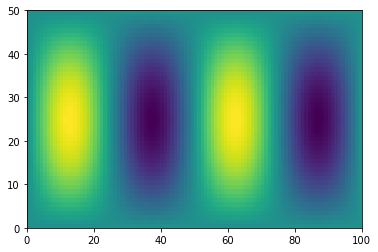## Manipulating array dimensions¶

In :
# transpose
plt.pcolormesh(f.T)

Out: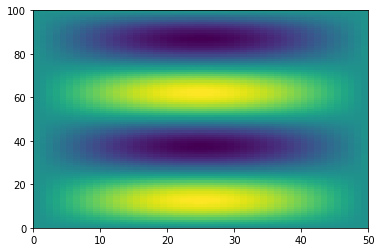In :
# reshape an array (wrong size)
g = np.reshape(f, (8,9))

---------------------------------------------------------------------------
ValueError                                Traceback (most recent call last)
in ()
1 # reshape an array (wrong size)
----> 2 g = np.reshape(f, (8,9))

/anaconda/lib/python3.6/site-packages/numpy/core/fromnumeric.py in reshape(a, newshape, order)
230            [5, 6]])
231     """
--> 232     return _wrapfunc(a, 'reshape', newshape, order=order)
233
234

/anaconda/lib/python3.6/site-packages/numpy/core/fromnumeric.py in _wrapfunc(obj, method, *args, **kwds)
55 def _wrapfunc(obj, method, *args, **kwds):
56     try:
---> 57         return getattr(obj, method)(*args, **kwds)
58
59     # An AttributeError occurs if the object does not have

ValueError: cannot reshape array of size 5000 into shape (8,9)
In :
# reshape an array (right size) and mess it up
print(f.size)
g = np.reshape(f, (200,25))
plt.pcolormesh(g)

5000

Out: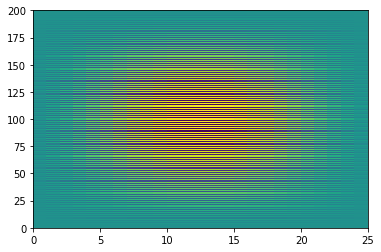In :
# tile an array
plt.pcolormesh(np.tile(f,(6,1)))

Out: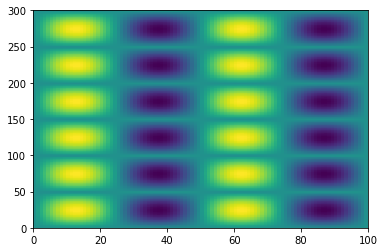## Broadcasting¶

Broadcasting is an efficient way to multiply arrays of different sizes

In :
Image(url='http://scipy-lectures.github.io/_images/numpy_broadcasting.png',
width=720)

Out:In :
# multiply f by x
print(f.shape, x.shape)
g = f * x
print(g.shape)

(50, 100) (100,)
(50, 100)

In :
# multiply f by y
print(f.shape, y.shape)
h = f * y
print(h.shape)

(50, 100) (50,)

---------------------------------------------------------------------------
ValueError                                Traceback (most recent call last)
in ()
1 # multiply f by y
2 print(f.shape, y.shape)
----> 3 h = f * y
4 print(h.shape)

ValueError: operands could not be broadcast together with shapes (50,100) (50,) 
In :
# use newaxis special syntax
h = f * y[:,np.newaxis]
print(h.shape)

(50, 100)

In :
plt.pcolormesh(g)

Out: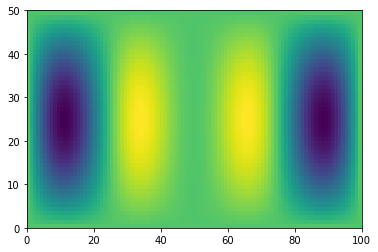## Reduction Operations¶

In :
# sum
g.sum()

Out:
-3083.0383878071548
In :
# mean
g.mean()

Out:
-0.61660767756143098
In :
# std
g.std()

Out:
1.6402280119141424
In :
# apply on just one axis
g_ymean = g.mean(axis=0)
g_xmean = g.mean(axis=1)

In :
plt.plot(x, g_ymean)

Out:
[]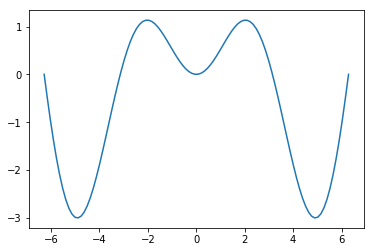In :
plt.plot(g_xmean, y)

Out:
[]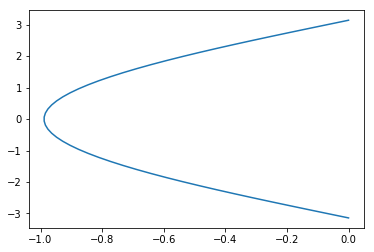## Fancy Plotting¶

Enough lessons, let's have some fun.

In :
fig = plt.figure(figsize=(12,8))
ax1 = plt.subplot2grid((6,6),(0,1),colspan=5)
ax2 = plt.subplot2grid((6,6),(1,0),rowspan=5)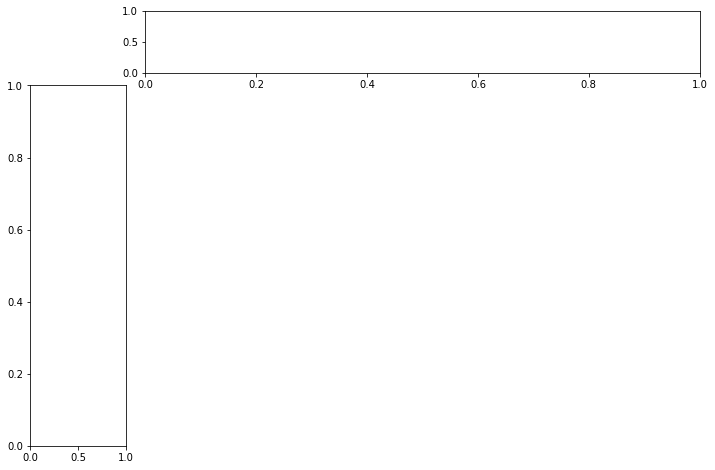In :
fig = plt.figure(figsize=(10,6))
ax1 = plt.subplot2grid((6,6),(0,1),colspan=5)
ax2 = plt.subplot2grid((6,6),(1,0),rowspan=5)
ax3 = plt.subplot2grid((6,6),(1,1),rowspan=5, colspan=5)

ax1.plot(x, g_ymean)
ax2.plot(g_xmean, y)
ax3.pcolormesh(x, y,  g)

ax1.set_xlim([x.min(), x.max()])
ax3.set_xlim([x.min(), x.max()])
ax2.set_ylim([y.min(), y.max()])
ax3.set_ylim([y.min(), y.max()])

plt.tight_layout()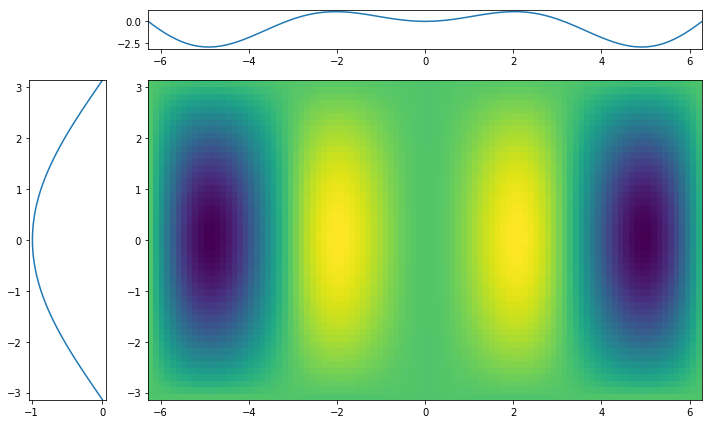## Real Data¶

ARGO float profile from North Atlantic

In :
# download with curl
!curl -O http://www.ldeo.columbia.edu/~rpa/argo_float_4901412.npz

  % Total    % Received % Xferd  Average Speed   Time    Time     Time  Current
Dload  Upload   Total   Spent    Left  Speed
100  140k  100  140k    0     0   402k      0 --:--:-- --:--:-- --:--:--  405k

In :
# load numpy file and examine keys
data = np.load('argo_float_4901412.npz')
data.keys()

Out:
['S', 'T', 'levels', 'lon', 'date', 'P', 'lat']
In :
# access some data
T = data['T']

In :
# there are "nans", missing data, which screw up our routines
T.min()

Out:
nan
In :
ar_w_mask = np.ma.masked_array([1, 2, 3, 4, 5],
mask=[True, True, False, False, False])
ar_w_mask

Out:
masked_array(data = [-- -- 3 4 5],
mask = [ True  True False False False],
fill_value = 999999)
In :
ar_w_mask.mean()

Out:
4.0
In :
T_ma = np.ma.masked_invalid(T)
T_ma.mean()

Out:
11.104955983298781

## Masked Arrays¶

This is how we deal with missing data in numpy

In :
# create masked array
T = np.ma.masked_invalid(data['T'])
type(T)

Out:
numpy.ma.core.MaskedArray
In :
# max and min
T.max(), T.min()

Out:
(24.364999771118164, 3.5320000648498535)
In :
# load other data
S = np.ma.masked_invalid(data['S'])
P = np.ma.masked_invalid(data['P'])

In :
# scatter plot
plt.scatter(S, T, c=P)
plt.grid()
plt.colorbar()

Out: